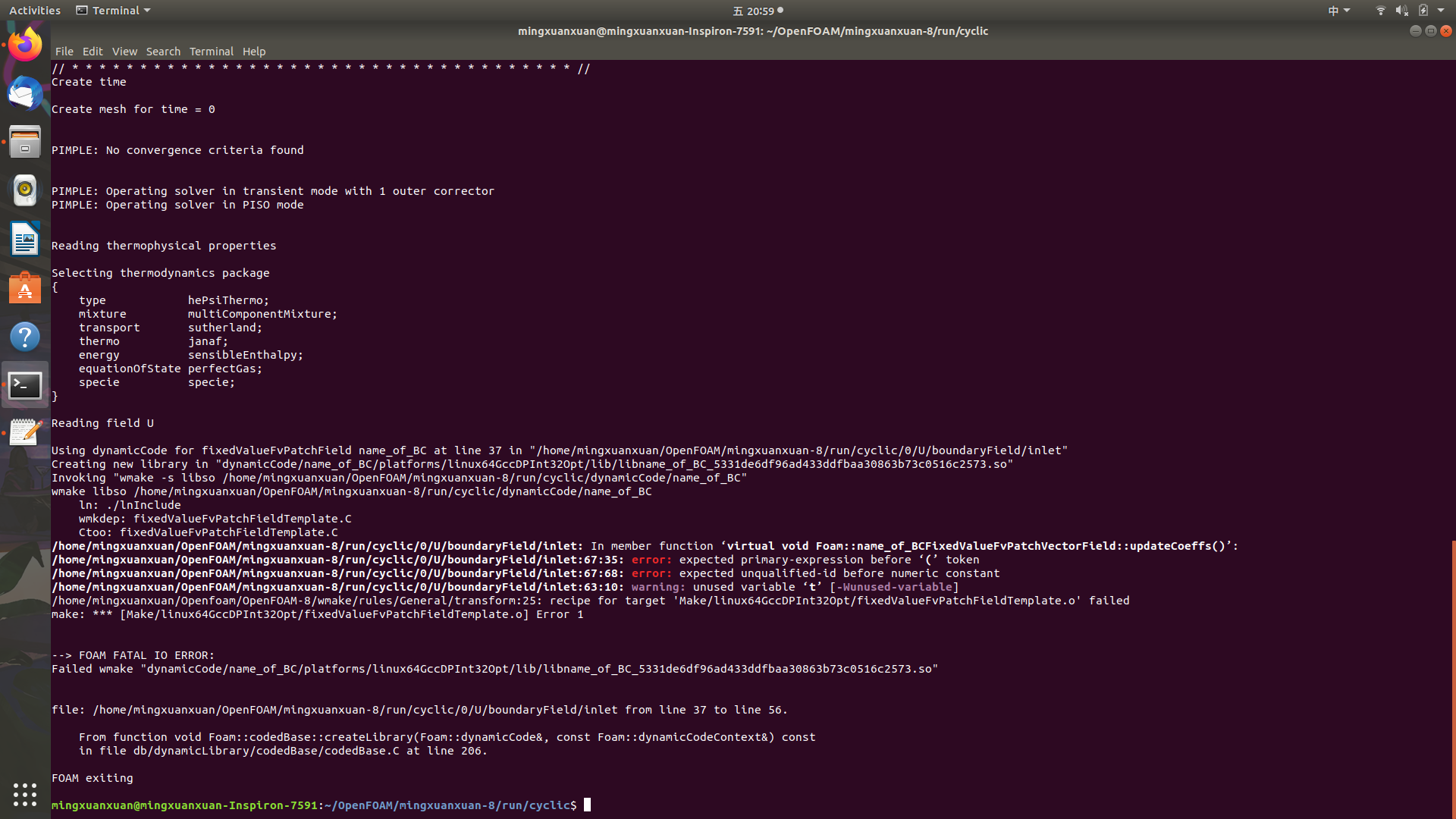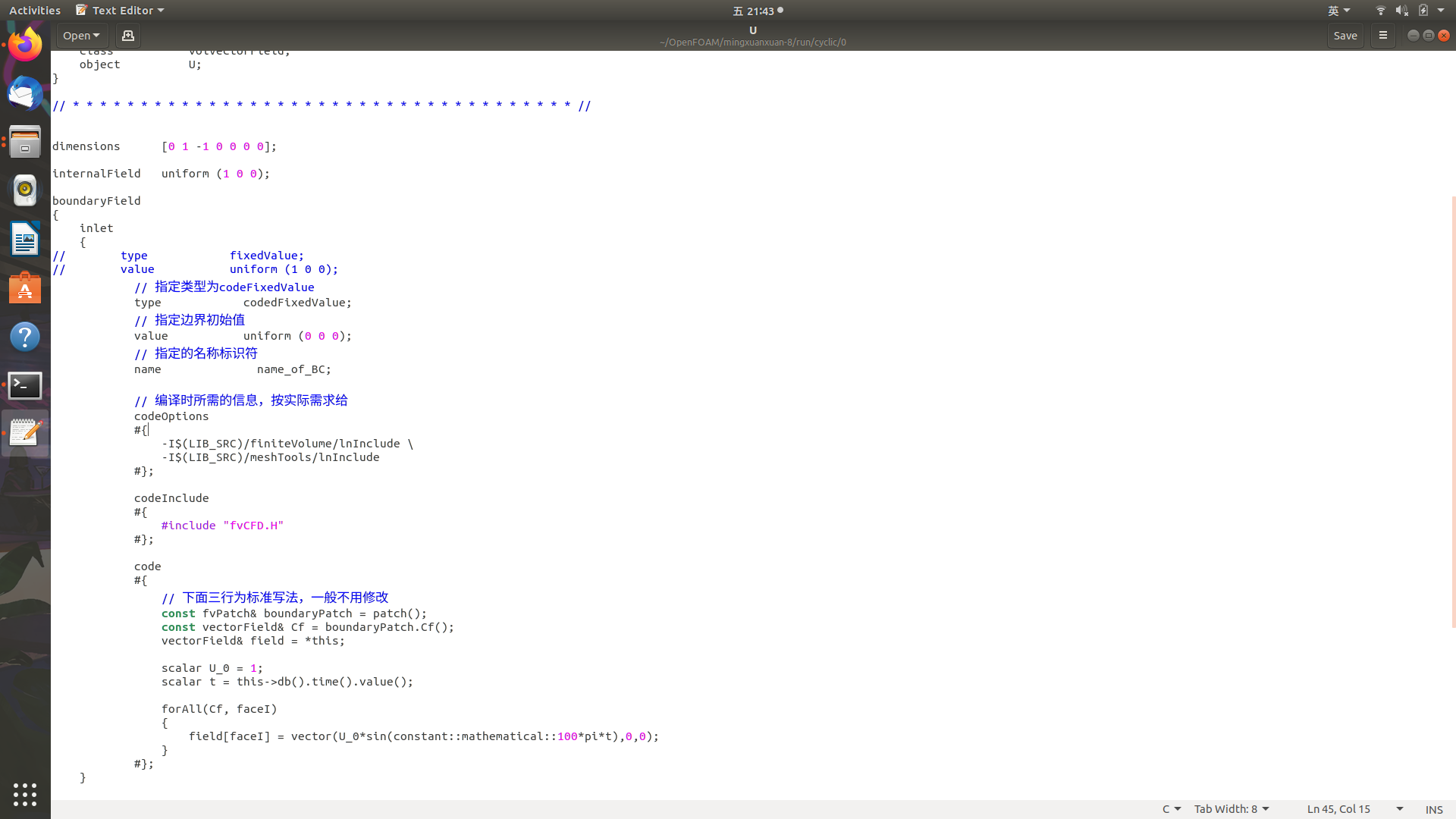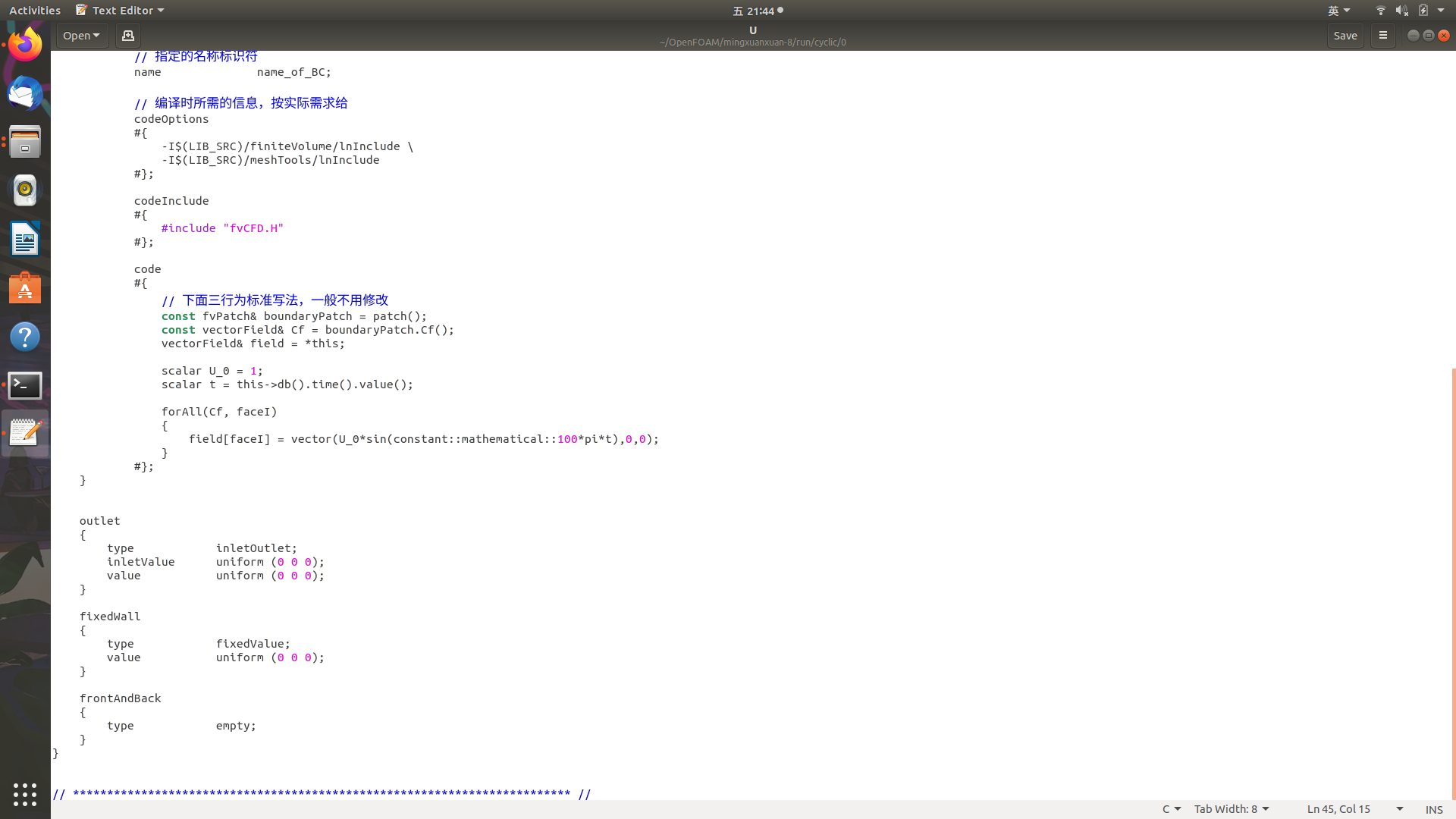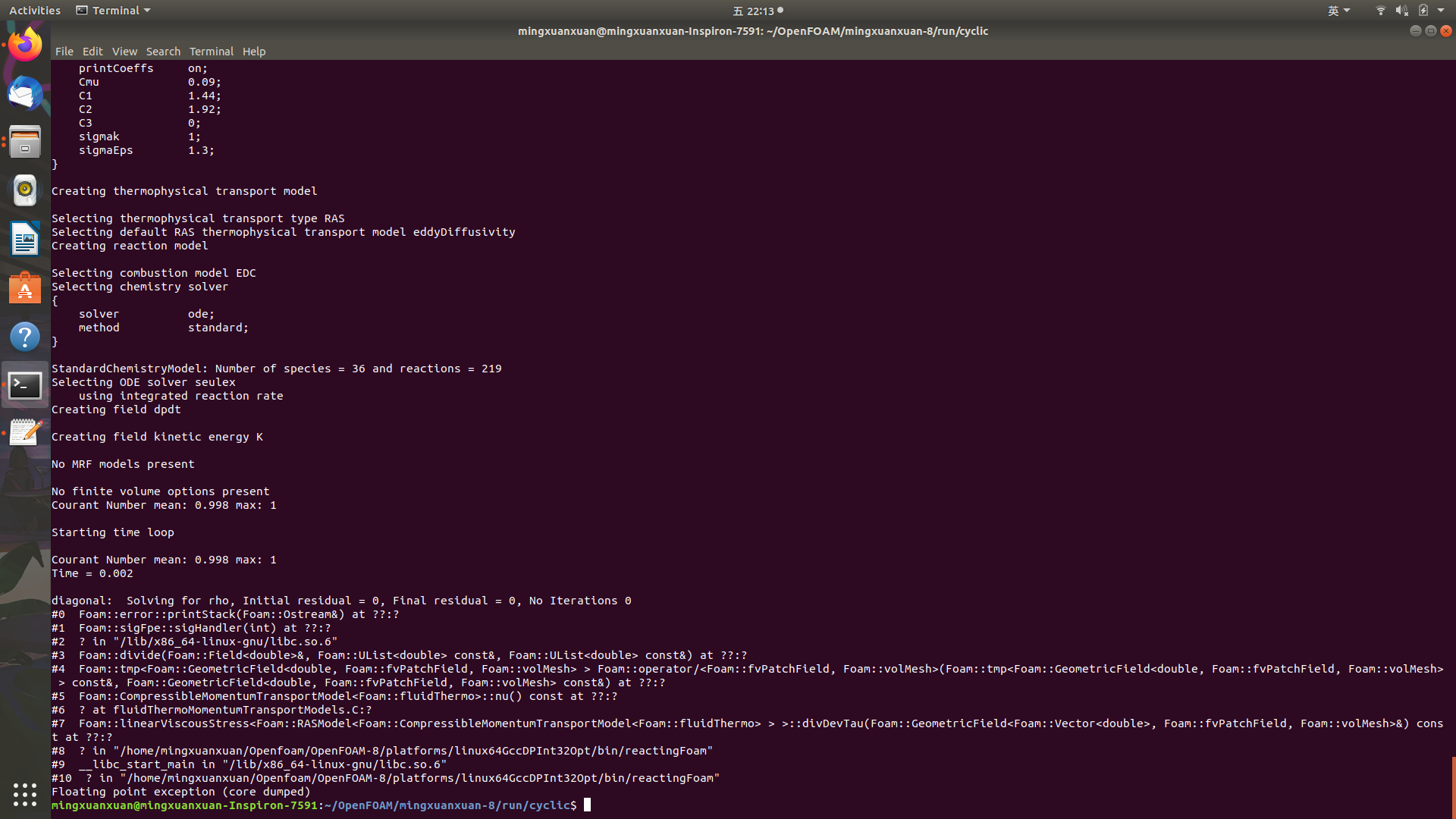# 关于cyclic boundary condition的一些问题

• 各位大佬好，本人小白在研究氢氧湍流燃烧时需要修改入口边界条件，想要设置速度随时间变化的正弦函数，经过研究我认为可以使用cyclic ，但不太清楚需要修改哪些文件，或者是否有大佬知道其他的非cyclic设置方式？之前有帖子说使用0/文件夹下的U文件进行设定，代码是db（）.time() .value()，但我不是很清楚代码的具体含义以及修改方式，是否有大佬能够点拨一二～

• 我是搬运工，仅供参考。
代码显示的问题，-I$$(LIB_SRC)/finiteVolume/lnInclude \ 应该改为： -I(LIB_SRC)/finiteVolume/lnInclude \  inlet { // type fixedValue; // value uniform (10 0 0); // 指定类型为codeFixedValue type codedFixedValue; // 指定边界初始值 value uniform (0 0 0); // 指定的名称标识符 name name_of_BC; // 编译时所需的信息，按实际需求给 codeOptions #{ -I$$(LIB_SRC)/finiteVolume/lnInclude \
-I\$(LIB_SRC)/meshTools/lnInclude
#};

codeInclude
#{
#include "fvCFD.H"
#};

code
#{
// 下面三行为标准写法，一般不用修改
const fvPatch& boundaryPatch = patch();
const vectorField& Cf = boundaryPatch.Cf();
vectorField& field = *this;

scalar U_0 = 10;
scalar t = this->db().time().value();

forAll(Cf, faceI)
{
field[faceI] = vector(U_0*sin(constant::mathematical::pi*100*t),0,0);
}
#};
}


• @wangfei9088 谢谢大佬，可是在跑的过程中还是有一些报错耶。• @明小米 方便把你的U文件贴出来吗？

• @wangfei9088是这样的。

• @明小米
你的pi那一行应该是写错了，100和pi写反了。
正确的应该是：

	            field[faceI] = vector(U_0*sin(constant::mathematical::pi*100*t),0,0);


这句constant::mathematical::pi可以这么理解：
常数::数学::圆周率

• @wangfei9088 请问大佬这是指u=sin100pi t吗？如果只用u=sint该如何表达呢？改了之后还是有报错耶• @明小米
对。就是u=sin(100pit)的意思，是我对pitzDaily那个case自己改的。

1. u=sint可以这么写：
	        scalar U_0 = 1;
scalar t = this->db().time().value();

forAll(Cf, faceI)
{
field[faceI] = vector(U_0*sin(t),0,0);
}

1. 最后那个报错：浮点数例外（核心已转储），可能的原因是哪个位置把0作为分母了。

• @wangfei9088 好的 报错的问题解决了 是由于初始速度设定为0了，修改为1就不报错了，非常感谢大佬！

• @wangfei9088 大佬 如果要改称sinx的绝对值应该如何修改代码呢？这里的速度代码似乎与C++的表示有些不同

• @明小米
两种方法吧，第一种平方再开方，第二种就是用mag(sin(t))表示求模：

	        scalar U_0 = 1;
scalar t = this->db().time().value();

forAll(Cf, faceI)
{
field[faceI] = vector(U_0*mag(sin(t)),0,0);
}


• @wangfei9088 好的，非常感谢大佬！